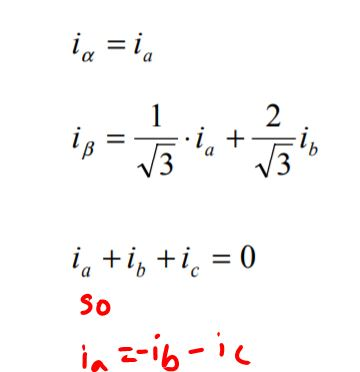# How does ODrive do current control?

#1

Hey, so I was looking at the schematics and the code and there is one thing that I still don’t quite understand. Maybe I just overlooked something.

ODrive seems to only measure current on two phases. Then it calculates Ialpha and Ibeta in some way. I don’t see how you can get every current vector just from measuring two low side phase currents.

What happens if we actually are between two vectors in such a way, that all the current flows through phase A? We would measure 0A at phase B and C.

Hope someone can explain that to me. Thanks!

#2

Hello MazzMan,
I think ODrive assumes perfectly balanced system in which the sum of currents in each phases are zero (courtesy of TI):I don’t quite understand this part of the question though:

``````What happens if we actually are between two vectors in such a way, that all the current flows through phase A? We would measure 0A at phase B and C.
``````

I’m also curious about these stuff so I’m posting in case it helps…

#3

Yes you are right! After doing some more research, this is just what the Clark Transformarion does. That means we only need to measure current on two phases.

The second question was badly formulated. All I meant was: how can we measure phase current using only a low side shunt resistor? I think it works due to the fact, that the current in an inductance cannot change instantaniously. When the high side transistor closes, the phase current will flow through the low side fly back diode and thus through the shunt resistor. Not sure if this is true.